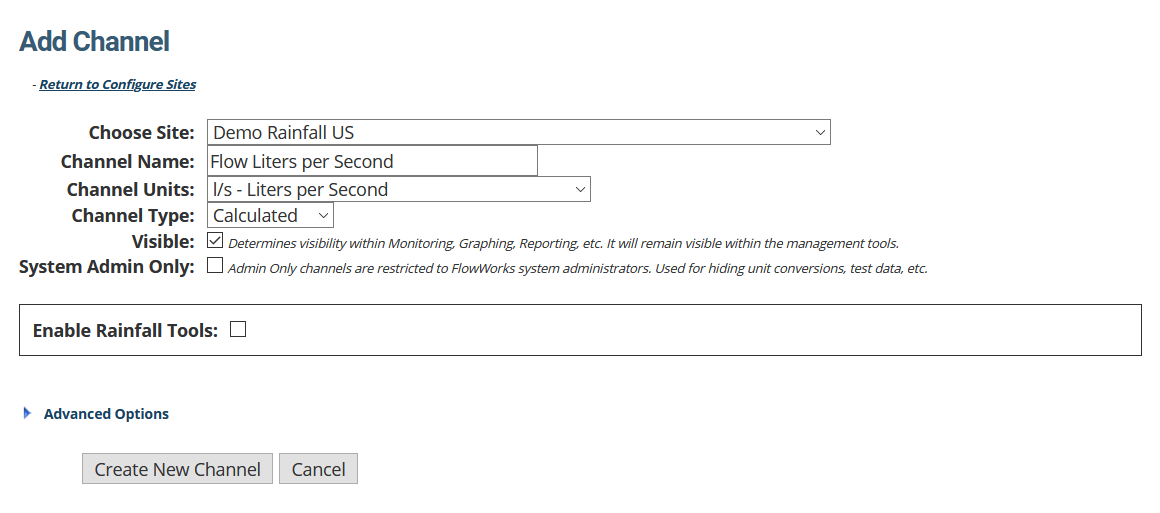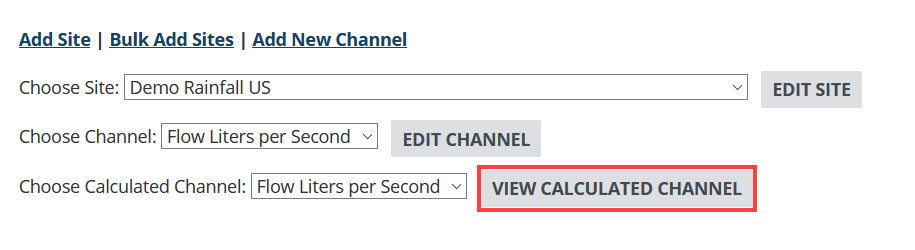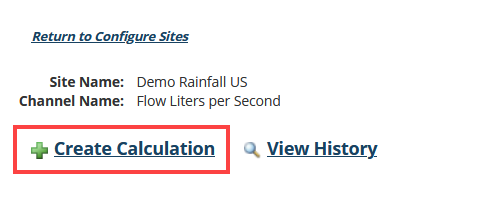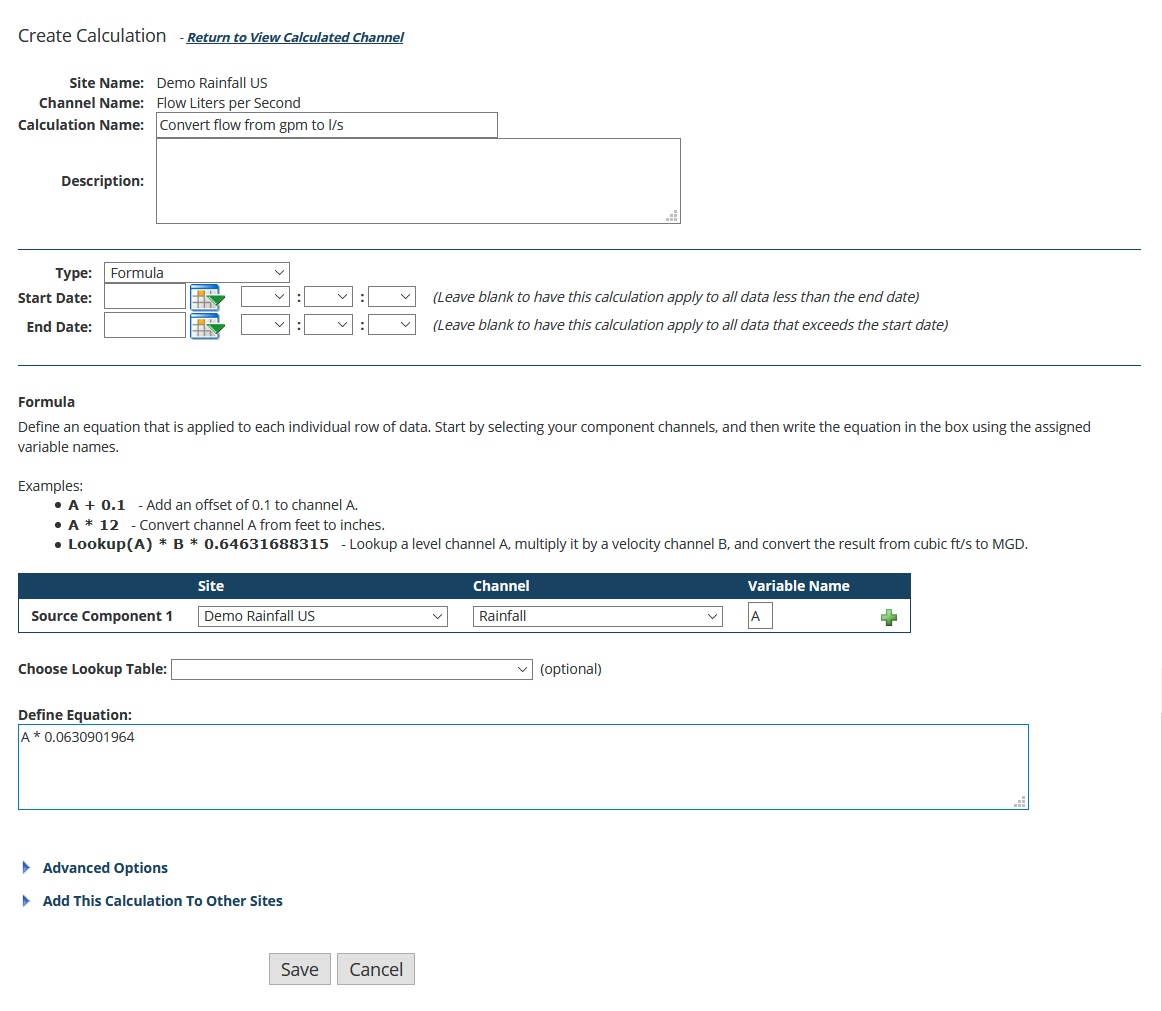# Formula Example: Convert Measurement Units of a Channel

Is your data in the wrong units? FACE can fix that.

You may find that an instrument in the field is sending Imperial units when you need it in Metric, or vice versa. You could send a tech out to change the units the instrument is reporting in, if the instrument is capable of sending data in the desired measurements. But then you will have a stream of data that suddenly changes from one measurement standard to another. In this article we’ll look at creating a calculated channel and applying a conversion, to the correct units, without requiring a trip to the field.

In this example, a user has an instrument sending flow in gallons per minute (gpm), but they want to see the flow in liters per second (l/s).

## The process for converting channel measurement units is twofold

1. Start by Creating a Calculated Channel. Once complete, you will need to proceed to step 2, which is discussed in this article. The image below shows channel setup for this example.1. Configure a FACE formula calculation that commands the system to simply copy the data from the original channel (see below).

This article is a continuation from the article, Create a Calculated Channel. From step one, it is assumed that you are back at the Configure Sites Page, after having completed setup of a new calculated channel.

## 1. Open the calculated channel you want to convert

1. Use the Choose Site dropdown locate the site that contains the channel with the calculation you wish to delete. The page will refresh, revealing channels and calculated channels under this site.
2. Use the Choose Calculated Channel dropdown to select the channel that contains the calculation you wish to delete.
3. Click View Calculated Channel.## 2. Click Create Calculation

This will take you to the page where you will configure the calculation.## 3. Configure the conversion formula

This page has three distinct sections - the following steps take you through each part, beginning from the top of the page.1. Provide a Calculation Name and (optional) Description.
2. Select Formula for Calculation Type.
3. Ignore Start Date and End Date.
We want this channel to be filled with data points for the entire data stream we have available.
4. Choose the Source Component.
This is going to be the original Flow channel that contains data we want to convert.
5. Leave Choose Lookup Table blank.
6. Define the equation. This requires that you ensure the conversion constant, which is circumstantial. For this example, we will use the equation A * 0.0630901964, the constant for conversion between gallons per minute (gpm) and liters per second (l/s).

## 4. Click Save

Now, you can use the Flow (l/s) Channel in lieu of the Flow (gpm) channel for graphing, reporting, alarming, etc. No trip to the field needed, no data stream suddenly changing units - just one nice stream of data, all in your desired units.